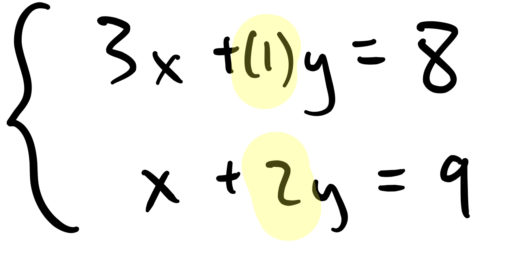In this post, we will talk about how to solve a system of linear equations via the method of elimination. Now, you will not need to know both this method and the method of substitution that we discuss in the next post. You do need to be able to solve a system of linear equations, but either method suffices for that purpose. However, for some problems, one or the other method may be easier. So if you have the time, it is worth learning both.

Now suppose we have a system of equations as follows:

ExampleWe could try to solve this system by guessing values ofand, and plugging them in to see if they work. But that would take a long time. Instead, note that if we subtract the second equation from the first, we get:Or, in other words,Now that we have found the value of, we can plug it back in to find the value of:So our solution is.

In general, the strategy for solving equations by elimination is to try and get some variables to cancel each other out. We ultimately want one line of the equation to have just one variable in it, for then it is easy to calculate the value of that variable. Once we have that value, we simply plug it back into an earlier equation to get the value of the remaining variable(s).

Let’s look at another example:

ExampleHere, it is not useful to simply subtract the second equation from the first. Doing so yields:which is no more helpful than what we started with.

Instead, we need to make a decision: do we want to eliminateor? It does not matter too much which one you choose (at most you can save yourself a few lines of arithmetic). Let’s say we want to eliminate. Then, we need to manipulate our equations so that thevariable will be eliminated. We see that in our second equation,has a coefficient ofbut in the first equation,only has a coefficient of.Subtracting these equations, then, will get us an equation that includes. To get the’s to cancel out, we needto have the same coefficient in both equations. So let’s multiply the first equation by. (We could have also divided the second equation by 2, but fractions are more annoying to work with). Then, we getAnd now, we can subtract the second equation from the first to get:Plugging this back into one of our initial equations, we get:So our solution isIn general, elimination problems will look like the following:whereare constants. Let’s lay out the steps for using the method of elimination in general:

Elimination Method

1. Pick eitherorto eliminate.

For our example, suppose we want to eliminate.

1. Forceto have the same coefficient in both equations.

To do that here, we multiply the first equation byto get:(The fact that we chose to manipulate the first equation is unimportant. We could have instead multiplied the second equation byto get similar results.)

1. Finally, we subtract the second equation from the first (or the first from the second, you can pick whichever is easier to calculate).

Here, we get:And while this looks rather complicated, recall thatare all just constants. So finding the value ofis now just a matter of plugging in some numbers into a calculator.

1. Once we know the value of, we can just plug it in to get the value of.

Here,.

Now, we can also use the method of elimination to solve systems of 3 equations. Here, the trick is to turn our system of 3 equations into a system of 2 equations. This is done by picking some variable to eliminate, and then applying (roughly) the above method to eliminate it. Then, we apply the above steps method in order to solve the remaining system of 2 equations. Here is an example of such a problem.

ExampleSuppose we want to eliminate the variable. Then, we multiply our equations by constants so thatwill have the same coefficient in every equation. Since we want to avoid working with fractions, let’s try to makehave the coefficient of 6 in every equation. Then, we multiply the first equation by, the second one by 2, and the third one by 3 to get:Now, we need to cancel out the’s. How do we do this? Now, as before, there are many ways to cancel out the’s. The important thing is that we use all three equations in this process. (For an explanation of why this is important, see this post. Intuitively, if you don’t use all three equations, you are losing information). So we cannot simply subtract the second equation from the first and then subtract the first equation from the second.

In our case, we will simply subtract the second equation from the first, and then subtract the third equation from the second. This yields:And we can solve this using the normal method of elimination discussed earlier.

Like any mathematical ability, really becoming proficient with the method of elimination requires practice. Here are some problems to practice on.

Practice Problems

Solve the following systems of equations using the method of elimination

1.1.2.3.